#Finding Roots of Polynomials Graphically and Numerically#### Finding real roots graphically.

The real number x=a is a root of the polynomial f(x) if and only if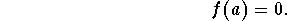When we see a graph of a polynomial, real roots are x-intercepts of the graph of f(x).

Let's look at an example: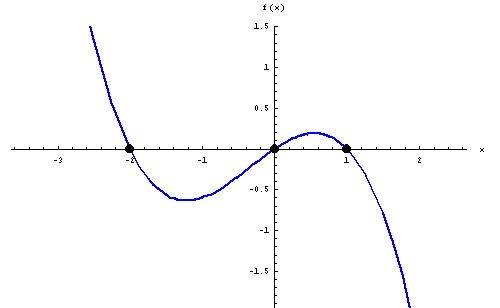The graph of the polynomial above intersects the x-axis at (or close to) x=-2, at (or close to) x=0 and at (or close to) x=1. Thus it has roots at (or close to) x=-2, at (or close to) x=0 and at (or close to) x=1.

The polynomial will also have linear factors (x+2), x and (x-1). Be careful: This does not determine the polynomial! It is not true that the picture above is the graph of (x+2)x(x-1); in fact, the picture shows the graph of f(x)=-.3(x+2)x(x-1).

Here is another example: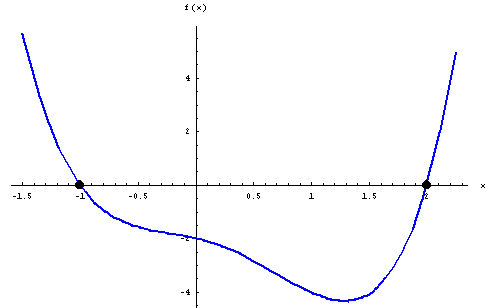The graph of the polynomial above intersects the x-axis at x=-1, and at x=2. Thus it has roots at x=-1 and at x=2.

The polynomial will thus have linear factors (x+1), and (x-2). Be careful: This does not determine the polynomial! It is not true that the picture above is the graph of (x+1)(x-2); in fact, the picture shows the graph of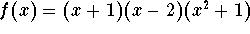. It is not even true that the number of real roots determines the degree of the polynomial. In fact, as you will see shortly,, a polynomial of degree 4, has indeed only the two real roots -1 and 2.

#### Finding real roots numerically.

The roots of large degree polynomials can in general only be found by numerical methods. If you have a programmable or graphing calculator, it will most likely have a built-in program to find the roots of polynomials.

Here is an example, run on the software package Mathematica: Find the roots of the polynomial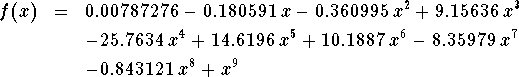Using the "Solve" command, Mathematica lists approximations to the nine real roots as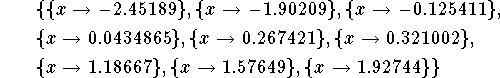Here is another example, run on Mathematica: Find the roots of the polynomial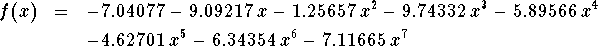Mathematica lists approximations to the seven roots as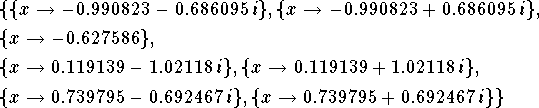Only one of the roots is real, all the other six roots contain the symbol i, and are thus complex roots (more about those later on). Note that the complex roots show up "in pairs"!

Numerical programs usually find approximations to both real and complex roots.

Numerical methods are error-prone, and will get false answers! They are good for checking your algebraic answers; they also are a last resort if nothing else "works".

#### Exercise 1.

Find approximations to the real roots of the polynomial f(x) depicted below.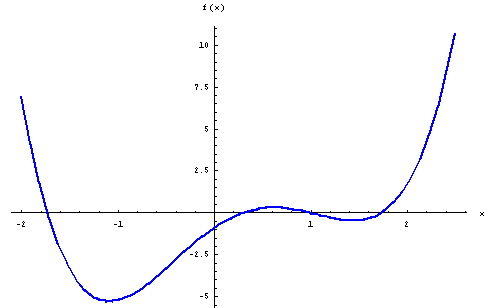#### Exercise 2.

What can you say about the degree of the polynomial f(x) depicted below?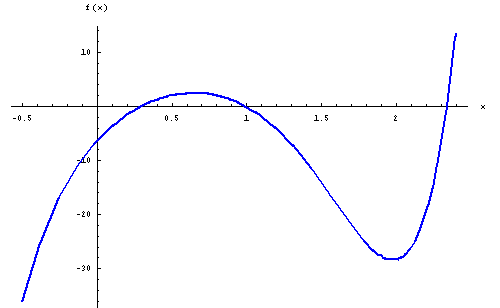#### Exercise 3.

If you have a device to compute roots numerically: Find all roots of the polynomial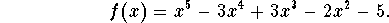How many real roots does the polynomial have?

#### Exercise 4.

If you have a device to compute roots numerically: Find all roots of the polynomial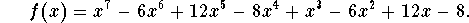How many complex roots does the polynomial have?[Back] [Next]
[Algebra] [Trigonometry] [Complex Variables]
[Calculus] [Differential Equations] [Matrix Algebra]S.O.S MATHematics home page

Do you need more help? Please post your question on our S.O.S. Mathematics CyberBoard.Helmut Knaust
Sun Jun 8 21:48:14 MDT 1997